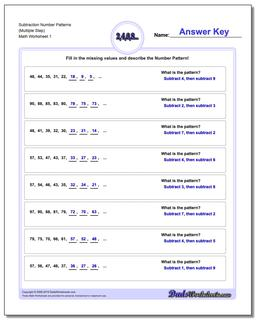# Math Worksheets: Number Patterns: Number Patterns: Subtraction Number Patterns (Multiple Step)## Subtraction Number Patterns (Multiple Step)

PropertyValue
DescriptionSubtraction Number Patterns (Multiple Step): Number pattern problems using only subtraction operations.
Resource TypeWorksheet